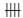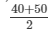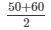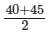# RD Sharma Solutions - Chapter 23 - Data Handling-I (Part - 2), Class 8, Maths Notes | Study RD Sharma Solutions for Class 8 Mathematics - Class 8

## Class 8: RD Sharma Solutions - Chapter 23 - Data Handling-I (Part - 2), Class 8, Maths Notes | Study RD Sharma Solutions for Class 8 Mathematics - Class 8

The document RD Sharma Solutions - Chapter 23 - Data Handling-I (Part - 2), Class 8, Maths Notes | Study RD Sharma Solutions for Class 8 Mathematics - Class 8 is a part of the Class 8 Course RD Sharma Solutions for Class 8 Mathematics.
All you need of Class 8 at this link: Class 8

PAGE NO 23.14:

Question 1:

The marks obtained by 40 students of class VIII in an examination are given below:
16, 17, 18, 3, 7, 23, 18, 13, 10, 21, 7, 1, 13, 21, 13, 15, 19, 24, 16, 3, 23, 5, 12, 18, 8, 12, 6, 8, 16, 5, 3, 5, 0, 7, 9, 12, 20, 10, 2, 23.
Divide the data into five groups, namely 0−5, 5−10, 10−15, 15−20 and 20−25 and prepare a grouped frequency table.

The frequency table for the marks of 40 students of class VIII in an examination is given below:

 Range of Marks Tally Marks Frequency 0−5|||| 9 5−10|||| 9 10−15|| 7 15−20|||| 9 20−25| 6

Question 2:

The marks scored by 20 students in a test are given below:
54, 42, 68, 56, 62, 71, 78, 51, 72, 53, 44, 58, 47, 64, 41, 57, 89, 53, 84, 57.
Complete the following frequency table:

 (Marks in class intervals) Tally marks Frequency (no. of children) 40−50 50−60 60−70 70−80 80−90

What is the class interval in which the greatest frequency occurs?

The frequency table can be completed as follows:

 Marks Tally Marks Frequency 40−50 |||| 4 50−60||| 8 60−70 ||| 3 70−80 ||| 3 80−90 || 2

The class interval with the greatest frequency (8) is 50−60.

Question 3:

The following is the distribution of weights (in kg) of 52 persons:

 Weight in kg Persons 30−40 10 40−50 15 50−60 17 60−70 6 70−80 4

(i) What is the lower limit of class 50−60?
(ii) Find the class marks of the classes 40−50, 50−60.
(iii) What is the class size?

(i) The lower limit of the class 50−60 is 50.
(ii) Class mark for the class 40−50:= 90/2 = 45
Again, class mark for the class 50−60:= 110/2 = 55
(iii) Here the class size is  40−30, i.e. 10.

Question 4:

Construct a frequency table for the following weights (in gm) of 35 mangoes using the equal class intervals, one of them is 40−50 (45 not included):
30, 40, 45, 32, 43, 50, 55, 62, 70, 70, 61, 62, 53, 52, 50, 42, 35, 37, 53, 55, 65, 70, 73, 74, 45, 46, 58, 59, 60, 62, 74, 34, 35, 70, 68.
(i) What is the class mark of the class interval 40−45?
(ii) What is the range of the above weights?
(iii) How many classes are there?

The frequency table for the given weights (in gm) of 35 mangoes is given below:

 Weight Tally Marks Frequency 30−40| 6 40−50| 6 50−60|||| 9 60−70|| 7 70−80|| 7

(i) Class mark for the class interval 40−45:
Class mark == 85/2 = 42.5
(ii) Range of the above weights:
Range = Highest value−Lowest value
= 74−30
= 44
(iii) There are 5 classes (30−40, 40−50, 50−60, 60−70, 70−80).

Question 5:

Construct a frequency table with class−intervals 0−5 (5 not included) of the following marks obtained by a group of 30 students in an examination.
0, 5, 7, 10, 12, 15, 20, 22, 25, 27, 8, 11, 17, 3, 6, 9, 17, 19, 21, 29, 31, 35, 37, 40, 42,45, 49, 4, 50, 16.

The frequency table with class intervals 0−5,5−10,10−15,...,50−55 is given below:

 Marks Tally Marks Frequency 0−5 ||| 3 5−105 10−15 ||| 3 15−205 20−25 ||| 3 25−30 ||| 3 30−35 | 1 35−40 || 2 40−45 || 2 45−50 || 2 50−55 | 1

Question 6:

The marks scored by 40 students of class VIII in mathematics are given below:
81, 55, 68, 79, 85, 43, 29, 68, 54, 73, 47, 35, 72, 64, 95, 44, 50, 77, 64, 35, 79, 52, 45, 54, 70, 83, 62, 64, 72, 92, 84, 76, 63, 43, 54, 38, 73, 68, 52, 54.
Prepare a frequency distribution with class size of 10 marks.

The frequency table of the marks scored by 40 students of class VIII in mathematics is given below:

 Mark Tally Marks Frequency 20−30 | 1 30−40 ||| 3 40−505 50−60||| 8 60−70||| 8 70−80|||| 9 80−90 |||| 4 90−100 || 2

PAGE NO 23.15:

Question 7:

The heights (in cm) of 30 students of class VIII are given below:
155, 158, 154, 158, 160, 148, 149, 150, 153, 159, 161, 148, 157, 153, 157, 162, 159, 151, 154, 156, 152, 156, 160, 152, 147, 155, 163, 155, 157, 153.
Prepare a frequency distribution table with 160−164 as one of the class intervals.

The frequency table is given below:

 Height Tally Marks Frequency 145−149 |||| 4 150−154|||| 9 155−159|| 12 160−1645

Question 8:

The monthly wages of 30 workers in a factory are given below:
830, 835, 890, 810, 835, 836, 869, 845, 898, 890, 820, 860, 832, 833, 855, 845, 804, 808, 812, 840, 885, 835, 836, 878, 840, 868, 890, 806, 840, 890.
Represent the data in the form of a frequency distribution with class size 10.

The frequency table of the monthly wages of 30 workers in a factory is given below:

 Wage Tally Marks Frequency 800−810 ||| 3 810−820 || 2 820−830 | 1 830−840||| 8 840−8505 850−860 | 1 860−870 ||| 3 870−880 | 1 880−890 | 1 890−9005

Question 9:

Construct a frequency table with equal class intervals from the following data on the monthly wages (in rupees) of 28 labourers working in a factory, taking one of the class intervals as 210−230 (230 not included):
220, 268, 258, 242, 210, 268, 272, 242, 311, 290, 300, 320, 319, 304, 302, 318, 306, 292, 254, 278, 210, 240, 280, 316, 306, 215, 256, 236.

The frequency table of the monthly wages of 28 labourers working in a factory is given below:

 Wage Tally Marks Frequency 210−230 |||| 4 230−250 |||| 4 250−2705 270−290 ||| 3 290−310|| 7 310−3305

Question 10:

The daily minimum temperatures in degrees Celsius recorded in a certain Arctic region are as follows:
−12.5,−10.8,−18.6,−8.4,−10.8,−4.2,−4.8,−6.7,−13.2,−11.8,−2.3, 1.2, 2.6, 0,−2.4, 0, 3.2, 2.7, 3.4, 0,−2.4,−2.4, 0, 3.2, 2.7, 3.4, 0,−2.4,−5.8,−8.9,−14.6,−12.3,−11.5,−7.8,−2.9
Represent them as frequency distribution table taking−19.9 to−15 as the first class interval.

The frequency table of the daily minimum temperatures is given below:

 Temperature Tally Marks Frequency −19.9 to −15 | 1 −14.9 to −10||| 8 −9.9 to −55 −4.9 to 0||| 13 0.1 to 5||| 8
The document RD Sharma Solutions - Chapter 23 - Data Handling-I (Part - 2), Class 8, Maths Notes | Study RD Sharma Solutions for Class 8 Mathematics - Class 8 is a part of the Class 8 Course RD Sharma Solutions for Class 8 Mathematics.
All you need of Class 8 at this link: Class 8Use Code STAYHOME200 and get INR 200 additional OFF

## RD Sharma Solutions for Class 8 Mathematics

88 docs

Track your progress, build streaks, highlight & save important lessons and more!

,

,

,

,

,

,

,

,

,

,

,

,

,

,

,

,

,

,

,

,

,

,

,

,

,

,

,

;